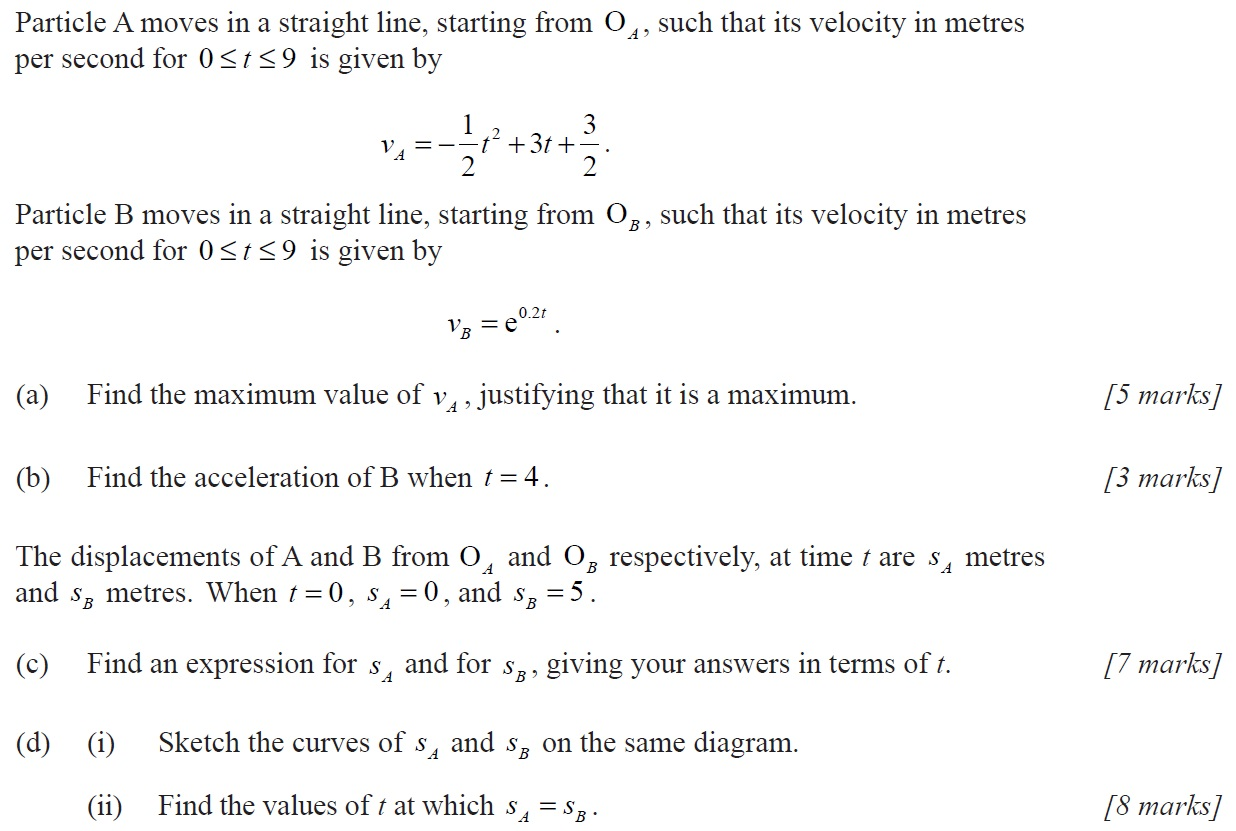# IBDP Past Year Exam Questions – Kinematics

1.[M00/P1]

The velocity,  $v$ , of an object, at a time  $t$ , is given by  $v=k{e}^{–\frac{t}{2}}$ , where  $t$ is in seconds and  $v$ is in m s-1. Find the distance travelled between $t=0$ and  $t=a$ .

2.[N13/P2/TZ0]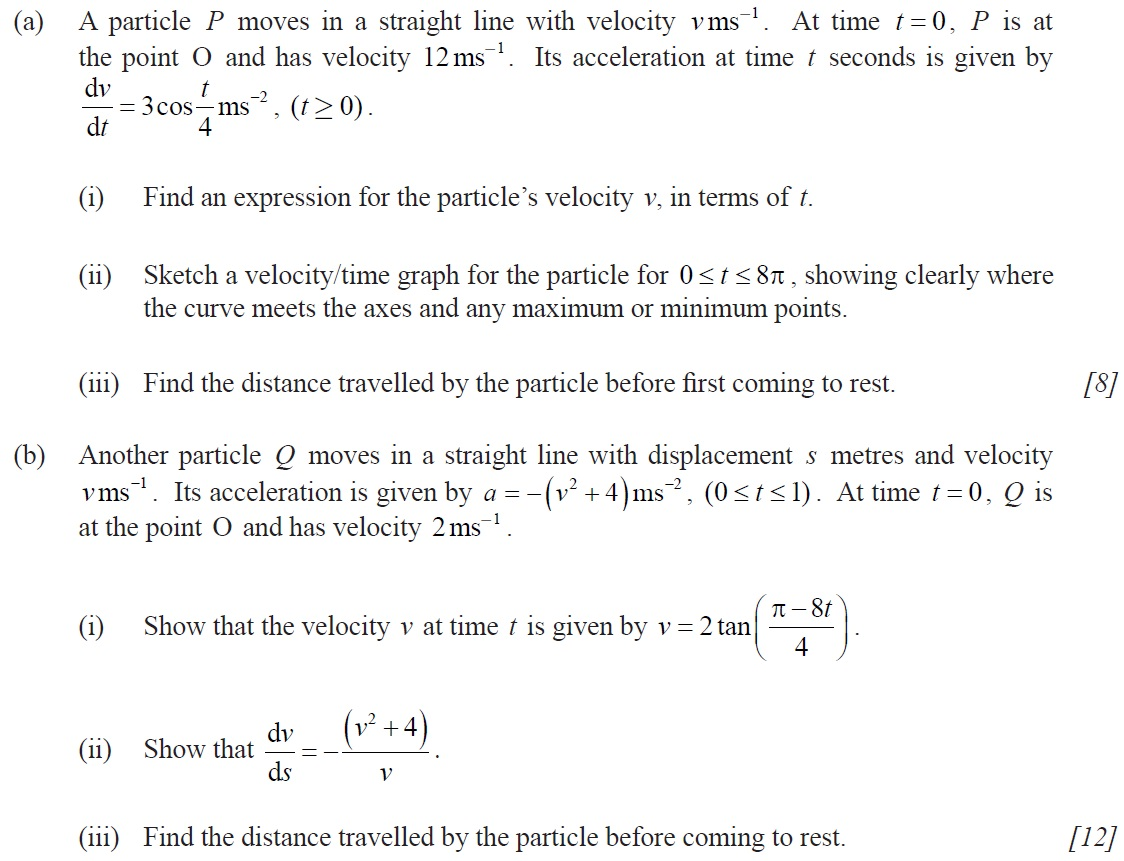3.[M14/P1/TZ1]

A body is moving in a straight line. When it is s metres from a fixed point O on the line its velocity, v , is given by  . Find the acceleration of the body when it is 50 cm from O.

4.[M15/P2/TZ1]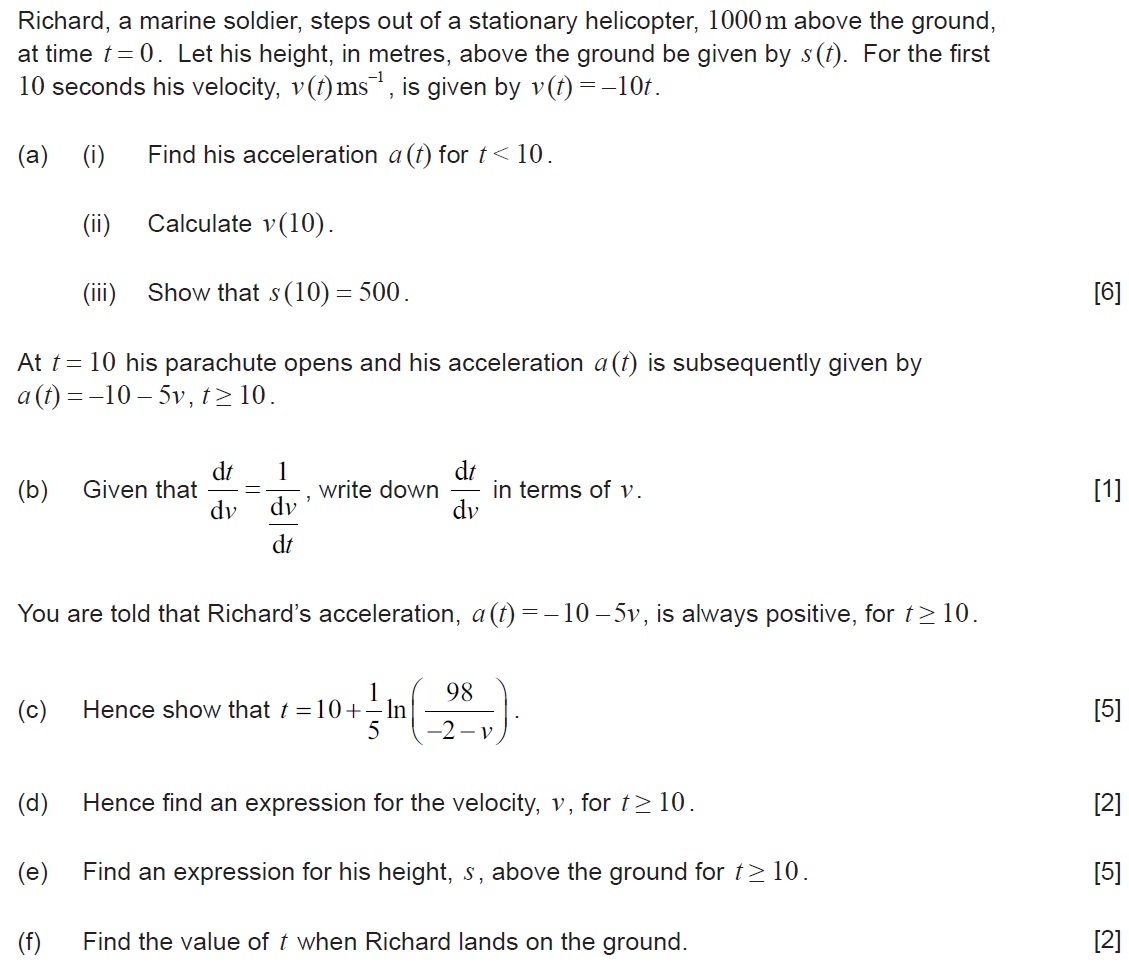5.[N15/P2/TZ0]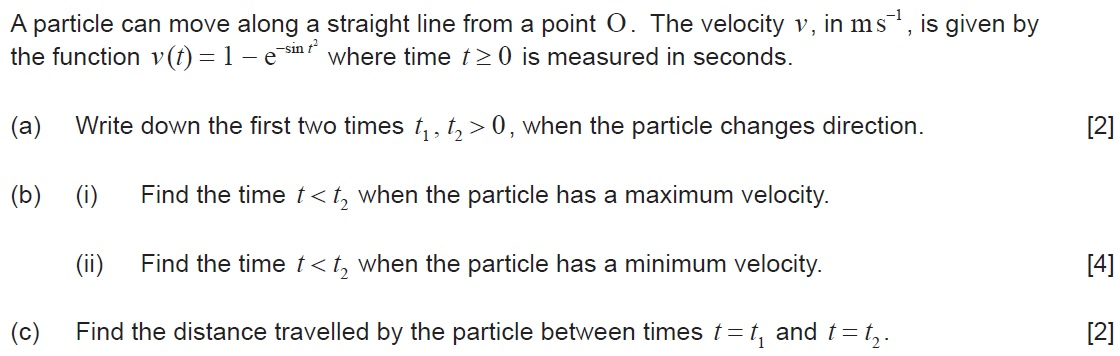6.[M17/P1/TZ2]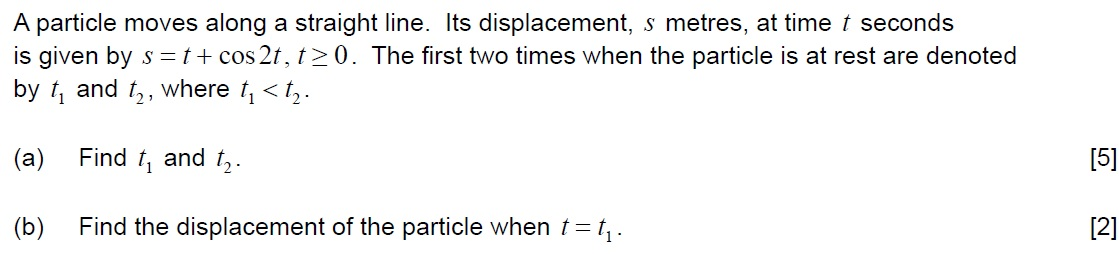7.[M06/P2/TZ0]